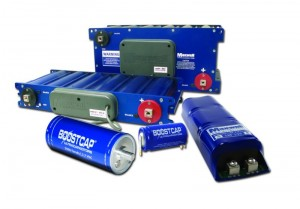Select Page

For anyone who would like to run their electric bike off of capacitors let me save you some time.

With current technology capacitors are not a viable option for powering an electric bike.

Currently, the energy density for ultra capacitors is at best about 1/10 that of lead acid batteries so it would take about 10 times the weight in ultra capacitors as it would for lead acid batteries.  Unless you have room for 300lb of capacitors this isn’t really an option.

A little wiki action:

(3–5 W·h/kg for a standard ultracapacitor, although 85 W.h/kg has been achieved in the lab as of 2010 compared to 30-40 W·h/kg for a lead acid battery)

Parameters Electrostatic Capacitor Ultracapacitor Battery
Discharge Time 10-6~10-3sec 1~30 sec 0.3~3 hrs
Charge Time 10-6~10-3sec 1~30 sec 1~5 hrs
Energy Density (Wh/kg) < 0.1 1~10 20~100
Power Density (W/kg) < 10,000 10,000 50~200
Charge / Discharge Efficiency ~ 1.0 ~10 0.7~0.85
Cycle Life Infinite > 500,000 500~2,000

http://www.nesscap.com/product/overview.jsp
http://en.wikipedia.org/wiki/Energy_density
http://en.wikipedia.org/wiki/Ultracapacitor

Even short trips require 4 or 5 amp hours of power at the rated voltage to be effective.

1 Farad is 1 Ampere second per Volt. 1 F = 1 As/V.
1 Ah = 3600 As
3600 As / 12V = 300 As/V = 300F
But you need more then that because the voltage will drop as the capacitor discharges. Half the charge means half the voltage.
With a lead acid battery that’s different. A battery that is half empty still has nearly the same voltage as it had at the start.
Maybe 600F and some electronics to automatically step up the voltage so it stays at 12V.
So you’d need to buy 160 capacitors with 3V and 60F each.Even after reading the above statement I decided to do more research and see if there is any new technology that could be useful. Ultra capacitors are starting to come down in price and are readily available, but they still are not cheap.
It is possible buy capacitors that are rated for 3000F and 2.7 volts, and their data sheets state that they have 3.30 Wh (~1.2Ah = 3.3Wh / 2.7V) of usable stored energy. The problem is that the calculation does not factor in the voltage drop that takes place as the capacitor discharges.

Ideally, with a bike you would want to run somewhere between 60 and 36 volts for a hub motor.

There are two options:
1. A voltage multiplier circuit.
2. A string of ultra capacitors in series.

The first idea is probably the better one, but it is fairly technical and goes beyond the scope of a blog post.
The basic math behind a series string of capacitors would be this. (Capacitance drops as capacitors are added in series.) 1/((1/3000)+(1/3000)+(1/3000)+(1/3000)+(1/3000)+(1/3000)+(1/3000)+(1/3000)+(1/3000)+(1/3000)+(1/3000)+(1/3000)+(1/3000)+(1/3000)+(1/3000)+(1/3000)+(1/3000)+(1/3000)) = 166. 7F

Theoretical storage would be
166. 7F*48V = 8000As
8000As/ 3600 As = 2.2 Ah

However, the usable potential is likely 1.2Ah which is stated on the data sheet, and the hub motor will operate most effectively between 48 and 36 volts. That is a 12V change and happens to provide access to about 25% of the usable 1.2 Ah in a simple series circuit.

……………………………. This is getting too boring.

If you still want to know more about sizing ultra capacitors for electric vehicles this article provides the required calculations. http://people.clarkson.edu/~pillayp/c1.pdf

Conclusion:
Start considering ultra capacitors for application in electric bikes  when ratings of 12000F + capacitors become available.  There were a lot of claims in 2007 and 2008 that we would see the end of batteries, but it looks like someone is getting close.

http://physicsworld.com/cws/article/news/44477